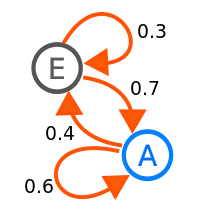# Graphical models¶

## Bayesian network¶

A directed acyclic graph where the nodes represent random variables.

Not to be confused with Bayesian neural networks.

### The chain rule for Bayesian networks¶

The joint distribution for all the variables in a network is equal to the product of the distributions for all the individual variables, conditional on their parents.wheredenotes the parents of the nodein the graph.

## Boltzmann Machines¶

### Restricted Boltzmann Machines (RBMs)¶

Trained with contrastive divergence.

## Clique¶

A subset of a graph where the nodes are fully-connected, ie each node has an edge with every other node in the set.

## Conditional Random Field (CRF)¶

Discriminative model that can be seen as a generalization of logistic regression.

Common applications of CRFs include image segmentation and named entity recognition.

### Linear Chain CRFs¶

A simple sequential CRF.

## Hidden Markov Model (HMM)¶

A simple generative sequence model in which there is an observable state and a latent state, which must be inferred.

At each time step the model is in a latent stateand outputs an observation. The observation is solely a function of the latent state, as is the probability distribution over the next state,. Hence the model obeys the Markov property.

The model is defined by:

• A matrixof transition probabilities whereis the probability of going from state i to state j.
• A matrixof emission probabilities whereis the probability of emitting observation j in state i.

The parameters can be learnt with the Baum-Welch algorithm.

## Markov chain¶

A simple state transition model where the next state depends only on the current state. At any given time, if the current state is node i, there is a probabilityof transitioning to node j, whereis the transition matrix.Source: Wikipedia

## Markov property¶

A process is said to have the Markov property if the next state depends only on the current state, not any of the previous ones.

## Markov Random Field (MRF)¶

A type of undirected graphical model which defines the joint probability distribution over a set of variables. Each variable is represented by one node in the graph.

One use for an MRF could be to model the distribution over the pixel values for a set of images. In order to keep the model tractable edges are only drawn between neighbouring pixels.

## Naive Bayes Model¶

A simple classifier that models all of the features as independent, given the label.